# High School Math : How to find the perimeter of a parallelogram

## Example Questions

### Example Question #1 : How To Find The Perimeter Of A Parallelogram

A parallelogram, with dimensions in cm, is shown below.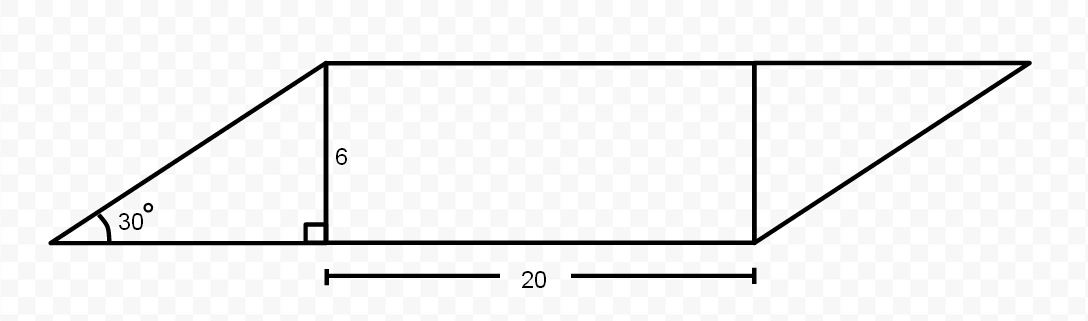What is the perimeter of the parallelogram, in cm?

Possible Answers: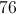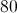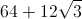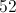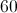Correct answer:Explanation:

The triangle on the left side of the figure has aand aangle. Since all of the angles of a triangle must add up to, we can find the angle measure of the third angle: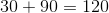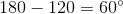Our third angle isand we have a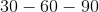triangle.

Atriangle has sides that are in the corresponding ratio of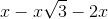. In this case, the side opposite ourangle is, so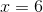We also now know that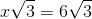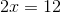Now we know all of our missing side lengths.  The right and left side of the parallelogram will each be. The bottom and top will each be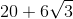. Let's combine them to find the perimeter: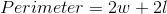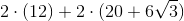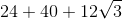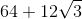### Example Question #24 : Quadrilaterals

Find the perimeter of the following parallelogram: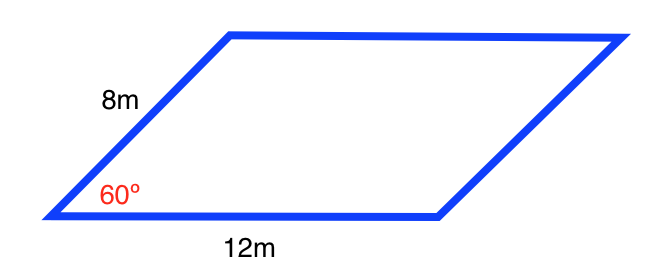Possible Answers: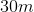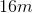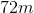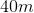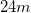Correct answer:Explanation:

The formula for the perimeter of a trapezoid is: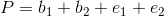,

whereis the length of the base and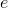is the length of the edge.

Opposite sides of a parallelogram have the same length. Therefore, both edges are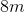and both bases are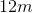.

Plugging in our values, we get: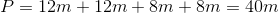### Example Question #25 : Quadrilaterals

Find the perimeter of the following parallelogram: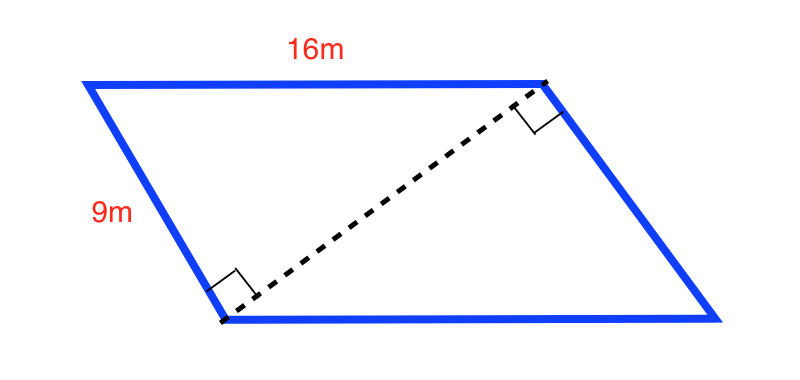Possible Answers: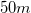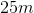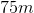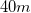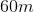Correct answer:Explanation:

The formula for the perimeter of a parallelogram is: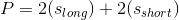where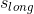is the length of the longer side and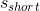is the length of the shorter side.

Plugging in our values, we get: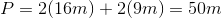### Example Question #26 : Quadrilaterals

Find the perimeter of the following parallelogram: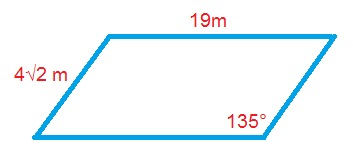Possible Answers: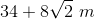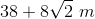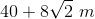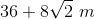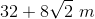Correct answer:Explanation:

The formula for the perimeter of a parallelogram is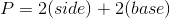.

Plugging in our values, we get: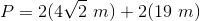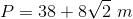### Example Question #1 : Parallelograms

ABCD is a parallelogram. BD = 5. The angles of triangle ABD are all equal. What is the perimeter of the parallelogram?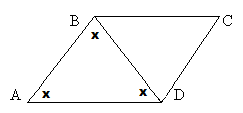Possible Answers: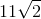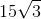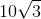Correct answer:Explanation:

If all of the angles in triangle ABD are equal and line BD divides the parallelogram, then all angles in triangle BDC must be equal as well.

We now have two equilateral triangles, so all sides of the triangles will be equal.

All sides therefore equal 5.

5+5+5+5 = 20

### All High School Math Resources